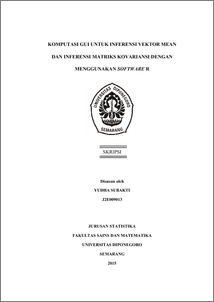# KOMPUTASI GUI UNTUK INFERENSI VEKTOR MEAN DAN INFERENSI MATRIKS KOVARIANSI DENGAN MENGGUNAKAN SOFTWARE R

SUBAKTI, YUDHA (2015) KOMPUTASI GUI UNTUK INFERENSI VEKTOR MEAN DAN INFERENSI MATRIKS KOVARIANSI DENGAN MENGGUNAKAN SOFTWARE R. Undergraduate thesis, FSM Universitas Diponegoro.Preview
PDF
1117Kb

## Abstract

Multivariate statistics is a branch of statistical science that discuss the analysis for multivariable case. Some analysis in multivariate statistics are discussing about inferences, there are inferences about mean vector and inferences of covariance matrices. Along with the development of technology, to support statistical analysis from both of inferences is requiring a statistical software, R is one of it with open source based. R is often used in statistical computing with command line interface (CLI) as the interface. In implementation, CLI requires the R user to remember names of used syntax and function. It makes less effective when the inferences have many related statistical analysis, so graphical user interface (GUI) needed to giving an easy way to accessing all of it. Testing for mean vectors of two populations will be performed using S. Rockiki’s data about measures of oxygen consumption for 25 males and 25 females. Results about assumptions showing both populations are normal multivariate distributed and have different covariannce matrix. The conclusion from the testing for mean vectors of two populations has performed is both populations have different mean vectors. There are packages are used on construction of GUI in R, including gdata, tcltk2, and devtools with additional software like Rtools and ActivePerl. The GUI has four main menus such as File, Analysis, Plot, and Help. Based on GUI usage, the GUI has been able to processing the chosen analysis and showing valid output. Keywords: Multivariate Statistics, Inferences about mean vector, Inferences of covariance matrices, R, GUI.

Item Type: Thesis (Undergraduate) H Social Sciences > HA Statistics Faculty of Science and Mathematics > Department of Statistics 47483 Mr Hasbi Yasin 02 Feb 2016 09:16 02 Feb 2016 09:16

Repository Staff Only: item control page